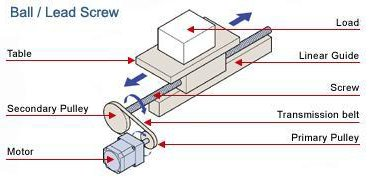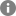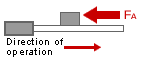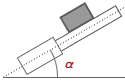Free Shipping for Online Orders. Terms and conditions apply.

## Ball / Lead Screw Application### Unit

Select the unit

Total weight mass of loads and table

W m

= lb kg

Friction coefficient of the guide

μ

=### Ball / Lead Screw Specifications

Diameter

DB

= in mm

Total length

LB

= in mm

PB

= in/rev mm/rev

(Distance the screw moves in one rotation)

Efficiency

η

=  %

ref; ballscrew 80 〜 95%,

Material

ρ

=

Breakaway torque of the screw

TB

= lb·in N·m

### External Force

FA

= lb N### Transmission Belt and Pulleys or Gears (Leave the fields blank if a direct coupling structure is used)

Primary pulley (gear) pitch circle diameter (PCD) or diameter

Secondary pulley (gear) pitch circle diameter (PCD) or diameter

Dp1

=   in mm

Dp2

=   in mm

Primary pulley (gear) weight mass

Secondary pulley (gear) weight mass

Wp1 mp1

=   lb kg

Wp2 mp2

=   lb kg

If you are not sure about the weight mass

If you are not sure about the weight mass

Primary pulley (gear) thickness

Secondary pulley (gear) thickness

Lp1

=   in mm

Lp2

=   in mm

Primary pulley (gear) material

Secondary pulley (gear) material

ρp1

=

ρp2

=

### Mechanism Placement

Mechanism angle

α

= °### Other Requirement(s)

It is necessary to hold the load even after the power supply is turned off.
→ You need an electromagnetic brake.

It is necessary to hold the load after the motor is stopped, but not necessary to hold after the power supply is turned off.

### Operating Conditions

 Fixed speed operation Operating speed V1 = in mm /s Acceleration/Deceleration t1 = s
 Variable speed operation Operating speed V1 = in mm /s 〜 V2 = in mm /s Acceleration/Deceleration t1 = s
 Positioning operation (Fill in the fields, if any)Rotor inertia JO = oz·in kg·m 2 Gear ratio i = If the rotor inertia and the gear ratio are unknown, the acceleration torque will be calculated with an inertia ratio of 5:1 (see the motor selection tips that will appear on the result window for the detail).Positioning distance L = in mm Positioning time t0 = s Stopping time ts = s If a specific acceleration / deceleration time is required t1 = s If a specific operating speed is required V = in mm /s If Positioning distance is given and acceleration/deceleration is unknown, it is calculated as one fourth of Positioing time.

### Stopping Accuracy

Stopping accuracy

±

in mm

### Safety Factor

Safety factor

The following is the estimated requirements. Please contact 1-800-468-3982 ( from overseas 1-847-871-5931 ) for assistance or questions.

### Sizing Results

JL

= [oz·in [kg·m 2]

Required Speed

Vm

= [r/min]

V2

= [r/min]

Required Torque

T

= [lb·in] = [oz·in] [N·m]

RMS Torque

Trms

= [lb·in] = [oz·in] [N·m]

Acceleration Torque

Ta

= [lb·in] = [oz·in] [N·m]

TL

= [lb·in] = [oz·in] [N·m]

Required Stopping Accuracy

Δθ

= [deg]

Other Requirement(s)

To print the calculation report, click    Full Report

To view the motor selection tips, click    Tips

×Call 1-800-GO-VEXTA(468-3982) or 1-847-871-5931Print

- given information -

Total weight mass of loads and table

W m

[lb] [kg]

Friction coefficient of the guide

μ

Diameter

DB

[in] [mm]

Total length

LB

[in] [mm]

PB

[in/rev] [mm/rev]

Efficiency

η

[%]

Material

ρ

[oz/in [kg/m 3]

Breakaway torque of the screw

TB

[lb·in] [N·m]

FA

[lb] [N]

### Transmission belt and pulleys or gears

Primary pulley (gear)

Secondary pulley (gear)

pitch circle diameter (PCD)

Dp1

= [in] [mm]

Dp2

= [in] [mm]

weight mass

Wp1 mp1

= [lb] [kg]

Wp2 mp2

= [lb] [kg]

thickness

Lp1

= [in] [mm]

Lp2

= [in] [mm]

material

ρ

= [oz/in [kg/m 3]

ρ

= [oz/in [kg/m 3]

Mechanism angle

α

= [°]

### Other requirement(s)

Is it necessary to hold the load even after the power supply is turned off?

Is it necessary to hold the load after the motor is stopped, but not necessary to hold after the power supply is turned off?

### Operating conditions

Fixed speed operation

Operating speed

V1

=

[in/s] [mm/s]

Acceleration / deceleration time

t1

=

[s]

### Operating conditions

Variable speed operation

Operating speed

V1

=

[in/s] [mm/s]

V2

=

[in/s] [mm/s]

Acceleration / deceleration time

t1

=

[s]

### Operating conditions

Positioning operation

Rotor inertia

JO

=

[oz·in kg·m 2]

Gear ratio

i

=

Positioning distance

L

=

[in] [mm]

Positioning time

t0

=

[s]

Stopping time

ts

=

[s]

Acceleration / deceleration time

t1

=

[s]

Specified speed

V

=

[in/s] [mm/s]

### Stopping accuracy

Stopping accuracy

Δl

= [in] [mm]

### Safety factor

Safety factor

S·F

=

- calculated result -

JW

=   W × 16 × ( PB / 2π )2 m ×( (PB×10-3 ) / 2π )2

=   × 16 × ( ( ×10-3)  / ( 2 × 3.14 ))2

= [oz·in [kg·m 2]

JS

=  ( π / 32 ) ρ ( LB ×10-3) ( DB ×10-3) 4

=  ( 3.14 / 32 ) ×  × ( ×10-3)  × ( ×10-3) 4

= [oz·in [kg·m 2]

JDp1

=  ( 1 / 8 ) wp1 × 16 × Dp1 mp1 × (Dp1×10-3) 2

=   ( 1 / 8 ) ×  × 16 × ( ×10-3) 2

= [oz·in [kg·m 2]

JDp1

=   ( π / 32 ) ρ ( Lp1 ×10-3) ( Dp1 ×10-3) 4

=   ( 3.14 / 32 ) ×  × ( ×10-3)  × ( ×10-3) 4

= [oz·in [kg·m 2]

JDP2

=   ( 1 / 8 ) wP2 × 16 × DP2 mP2 × (DP2×10-3) 2

=   ( 1 / 8 ) ×  × 16 × ( ×10-3) 2

= [oz·in [kg·m 2]

JDP2

=  ( π / 32 ) ρ ( Lp2 ×10-3) ( Dp2 ×10-3) 4

=   ( 3.14 / 32 ) ×  × ( ×10-3)  × ( ×10-3) 4

= [oz·in [kg·m 2]

JL

=   ( JW + JS + JDp2 ) ( Dp1 / Dp2 )2 + JDp1

= (  +  +  ) × (  /  )2 +

[oz·in [kg·m 2]

JL

=  JW + JS

=  (  +  )

[oz·in [kg·m 2]

### Required Speed

 Vm =   V1 ( 60 / PB )   ( Dp2 / Dp1 ) =    × ( 60 /  ) × (  /  ) = [r/min]
 Vm1 =   V1 ( 60 / PB ) ( Dp2 / Dp1 ) =    × ( 60 /  ) × (  /  ) = [r/min] Vm2 =   V2 ( 60 / PB ) ( Dp2 / Dp1 ) =    × ( 60 /  ) × (  /  ) = [r/min]
 Vm =   (( L ( Dp2 / Dp1 ) ) / ( t0 - t1 )) ( 60 / PB ) = ((   × (  /  ) ) / (  -  )) × ( 60 /  ) = [r/min] Vm =   ( V / PB ) × 60 × ( Dp2 / Dp1 ) = (  /  ) × 60 × (  /  ) = [r/min]

### Required Torque

T

=   ( Ta + TL ) ( Safety Factor )

= (  +  ) ×

= [lb·in] [N·m]

= [oz·in]

### RMS Torque

Trms

=

√(((( Ta + TL )2 × t1 ) + ( TL2 × (t0 - 2 × t1 )) + (( Ta - TL )2 × t1 )) / ( t0 + ts )) × (Safety Factor)

=

√ ((((  +  )2 ×  ) + ( 2 × (  - 2 ×  )) + ((  -  )2 ×  )) / (  +  )) ×

= [lb·in] [N·m]

= [oz·in]

### Acceleration Torque

 Ta = ( JL / 386 ) ( Vm / ( 9.55 × t1 )) ( 1 / 16 ) = (   / 386 ) × (  / ( 9.55 ×  )) × ( 1 / 16 ) = [lb·in] [N·m] = [oz·in]
 Ta = ( JL / 386 ) ( Vm / ( 9.55 × t1 )) ( 1 / 16 ) = (  / 386 ) × (  / ( 9.55 ×  )) × ( 1 / 16 ) = [lb·in] [N·m] = [oz·in]
 Ta = (( JO i2 + 1.2 × JO + JL ) / 386 ) ( Vm / ( 9.55 × t1 )) ( 1 / 16 ) = ((  × 2 + 1.2 × +  )  / 386 )  × (   / ( 9.55 ×  ))  × ( 1 / 16 ) = [lb·in N·m] = [oz·in]

F

=   FA + W (m × 9.8) ( sinα + μcosα )

=    + (  × 9.8)  ( sin  +  × cos  )

[lb N]

TL

=   (((( F × PB ×10-3 ) / 2π ) × 1.1 ) +  TB ) ( Dp1 / Dp2 ) ( 1 / ( η × 0.01 ))

=   ((((  ×   ×10-3 ) / ( 2 × 3.14 )) × 1.1 ) +  ) × (  /  ) × ( 1 / (  × 0.01 ))

= [lb·in] [N·m]

=   [oz·in]

### Required Stopping Accuracy

Δθ

=  Δl ( 360° / PB ) ( Dp2 / Dp1 )

=    × ( 360 /  ) × (  /  )

[deg]

### Other requirement(s)

- end of the report -